# Implying the Probability of an EPA Reversal on the Write Down of the Renewable Mandate from the RINs Market

• Scott Irwin
• Department of Agricultural and Consumer Economics
• University of Illinois
March 5, 2014
farmdoc daily (4):41
Recommended citation format: Irwin, S. "Implying the Probability of an EPA Reversal on the Write Down of the Renewable Mandate from the RINs Market." farmdoc daily (4):41, Department of Agricultural and Consumer Economics, University of Illinois at Urbana-Champaign, March 5, 2014.

In a farmdoc daily post on February 19th, it was argued that the RINs market provided an early warning signal about another change in EPA policy.  More specifically, the rise of D6 ethanol prices relative to D4 biodiesel prices suggested that RINs traders believed the odds of the EPA reversing the proposed write down of the renewable mandate for 2014 in final rulemaking had increased sharply. The “coupling” of D4 and D6 RINs prices indicated a revised expectation that the renewable mandate for 2014 would exceed the E10 blend wall.  Figure 1, updated from the February 19th post, shows that D4 and D6 prices have continued to track one another, albeit with some erosion in D6 prices compared to D4 prices (about 5 cents). Analysis in another post on February 28th indicated that the big winner from a reversal of the write down of the renewable fuels mandate for 2014 was likely to be the biodiesel industry.  The reason is that biomass-based diesel was cheaper than E85 in most scenarios in terms of compliance costs for obligated parties under the RFS.  The purpose of today’s post is to drill down further and imply the probability of an EPA reversal by comparing current RINs values with the predictions from a simple joint probability model of RINs valuation.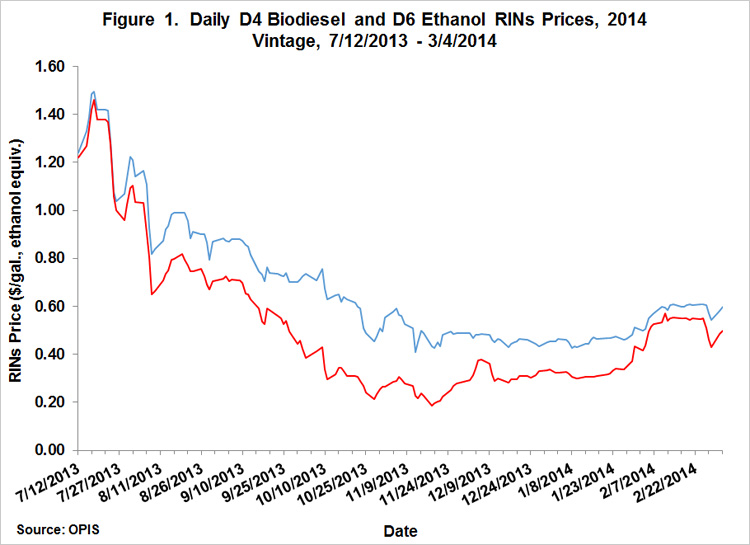The analysis makes use of the same 2 x 2 joint probability model used in two posts late last year (here and here) about RINs values.  This model predicts 2014 vintage RINs prices depending on the two main sources of policy uncertainty: i) the outcome of EPA rulemaking for 2014; and ii) the status of the biomass-based diesel blenders tax credits in 2014.  This situation can be illustrated for D4 biomass-based diesel RINs as follows: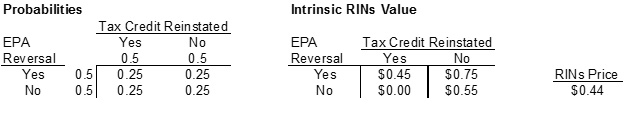The rows in the left panel represent the two possible outcomes of EPA policy regarding the renewable mandate for 2014, yes the write down is reversed or no the write down remains in place.  The columns represent the two possible outcomes for (retroactive) reinstatement of the blenders tax credit to 2014, yes it is reinstated and no it is not.  As a starting point for the analysis, equal probabilities are assumed in terms of yes/no for the reversal and reinstating the tax credit.  The four innermost cells in the left panel represent the four possible joint outcomes.  For example, the upper-left cell represents the outcome of the EPA reversing the write down and reinstatement of the tax credit for 2014.  Since the EPA and tax credit outcomes can be assumed to be independent events, it is easy to compute the joint probabilities for the four combinations of outcomes–simply cross-multiply the relevant yes/no probabilities for each cell.  Since the yes/no probabilities are all assumed in this example to be 0.5, the joint probabilities are all 0.25 (0.5 x 0.5).  This is a common assumption for probabilities if one assumes “diffuse” knowledge about the likely outcomes.  Also notice that the four joint probabilities sum to one.

The next step in the analysis is to determine the intrinsic RINs value for each of the four joint outcomes in the right panel.  Fortunately, those values can be obtained using the 2014 biomass-based diesel supply/demand model presented in the February 28th post.  Values for the bottom row in the right-hand panel can be obtained from the version of the supply/demand model found in Figure 2 of that post.  If the EPA does not reverse the renewable write down and the tax credit is reinstated, the supply/demand model predicted a RINs value of zero, which is the value used in the lower-left cell of the panel.  If the EPA dose not reverse the renewable write down and the tax credit is not reinstated, the same version of the supply/demand model predicted a RINs value of \$0.55 (0.82/1.5), which is the value used in the lower-right cell of the panel.  Values for the top row in the right hand panel can be found in a similar manner using Figure 3 and 4 from the February 28th post.

All that is left to do now is to multiply the joint probabilities by the intrinsic values to determine the “actuarially fair” or “expected” price for 2014 vintage D4 RINs today:

• \$0.44 = (0.25 x \$0.00) + (0.25 x \$0.55) + (0.25 x \$0.45) + (0.25 x \$0.75).

This set of assumptions predicts a D4 RINs price of \$0.44 per gallon (ethanol equivalents).  This price can be compared to 2014 vintage D4 RINs prices actually traded in the market during recent days, about \$0.55 to \$0.60 per gallon.  The calculated price from the joint probably model is substantially lower than actual prices, indicating that either the intrinsic RINs values in the right panel are too low or the assumption of equal probabilities is incorrect.  The table below presents the same joint probability analysis as above but instead assumes 80/20 probabilities of an EPA reversal: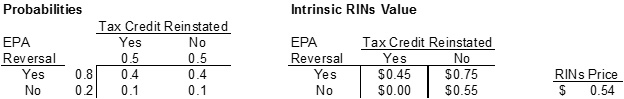The “actuarially fair” price for 2014 vintage D4 RINs today under these assumptions is:

• \$0.54 = (0.1 x \$0.00) + (0.1 x \$0.55) + (0.40 x \$0.45) + (0.40 x \$0.75).

This prediction is much closer to observed 2014 vintage D4 RINs prices at the present time and suggests the market is placing a substantial weight on the probability of an EPA reversal of the renewable mandate write down.

We can use the same joint probability model to predict the price of 2014 vintage D6 ethanol RINs as follows: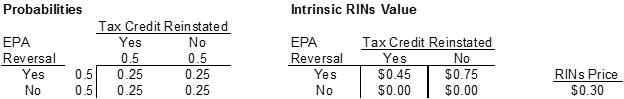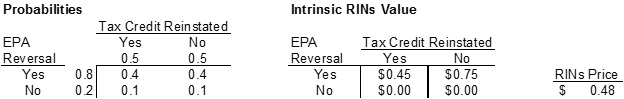Values for the bottom row in the right hand panels are zero reflecting an assumption that the EPA does not reverse the write down and positive blending margins for ethanol.  Values for the top row in the right-hand panel are the same as there are used to predict D4 biomass-based diesel RINs prices. This reflects the assumption that D4 and D6 prices are coupled when the renewable mandate exceeds the E10 blend wall, which would occur if the EPA write down is reversed.  The “actuarially fair” price for a 2014 vintage D6 RINs today under the assumption of equal probabilities is:

• \$0.30 = (0.25 x \$0.00) + (0.25 x \$0.00) + (0.25 x \$0.45) + (0.25 x \$0.75).

This price can be compared to 2014 vintage D6 RINs prices actually traded in the market in recent days, about \$0.45 to \$0.50 per gallon.  Once again, the calculated price from the joint probably model is substantially lower than actual prices under the assumption of equal probabilities.  If we instead assume 80/20 probabilities of an EPA reversal, the “actuarially fair” price for 2014 vintage D6 RINs today under these assumptions is:

• \$0.48 = (0.1 x \$0.00) + (0.1 x \$0.00) + (0.40 x \$0.45) + (0.40 x \$0.75).

This prediction is quite close to the observed D6 RINs prices at the present time and provides further confirmation that the market is placing a substantial weight on the probability of an EPA reversal of the renewable mandate write down.  Of course, this conclusion depends on the other assumptions used in the analysis, such as the price of soybean oil, the price of petroleum diesel, and a 50/50 chance of the biomass-based diesel blenders credits being reinstated.  Implied probabilities will change if these assumptions are altered.

#### Implications

RINs market prices currently imply about an 80 percent probability of a reversing the EPA’s write down of the renewable mandate for 2014 in final rulemaking.  Importantly, the probability calculation is fairly consistent across 2014 vintage D4 and D6 RINs prices.  Given the heated political battle surrounding the 2014 RFS rulemaking it is more than a bit surprising to see the RINs market leaning so heavily in the direction of a reversal. But this view received a boost recently from a prominent supporter of biofuels. Senator Charles Grassley of Iowa last week told reporters, “I would doubt there would be a complete reversal [from the proposal], which is what I would like to happen. I’d be surprised if there wasn’t some modification of the original rule that still might be considered a partial victory for ethanol.”  A public statement of this nature from Senator Grassley suggests the main uncertainty at this point is not whether the EPA will reverse the write down of the renewable mandate for 2014, but, rather, how much the write down will be rolled back.

Disclaimer: We request all readers, electronic media and others follow our citation guidelines when re-posting articles from farmdoc daily. Guidelines are available here. The farmdoc daily website falls under University of Illinois copyright and intellectual property rights. For a detailed statement, please see the University of Illinois Copyright Information and Policies here.